# Algebra II : Algebra II

## Example Questions

### Example Question #21 : Calculating Probability

If Gambit has a deck of 54 cards (a standard deck with two jokers), what is the probability that he will pick a queen followed by a joker? (Assume no replacement)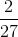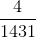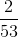Explanation:

If Gambit has a deck of 54 cards (a standard deck with two jokers), what is the probability that he will pick a queen followed by a joker? (Assume no replacement)

To calculate probability of multiple independent events, we need to calculate the probability of each event and multiply them together.

Recall that probability of an event can be found via the following: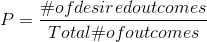So the probability of selecting a queen in the first pick is: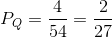And the probability of picking a joker in the second pick is: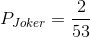So the probability of a queen followed by a joker is: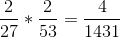Making our answer:### Example Question #22 : Calculating Probability

In a group of 150 Varsity Tutor students, 80 of them receive online tutoring, 63 of them receive in-person tutoring, 7 of them receive both, and 43 of them are tutored in math. What is the probability of choosing a random student that receives in-person tutoring in math?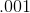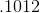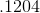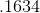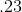Explanation:

In order to find the probability of these both happening, you need to find the probability of each event and then multiply them together.

The probability of picking an in-person tutoring student is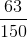The probability of picking a student who needs math tutoring is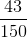To find the probability of both of these events happening you multiply: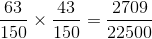or you can also do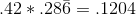### Example Question #21 : Calculating Probability

What is the probability of drawing a red ace in a standardcard deck?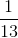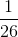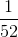Explanation:

We have four suits which are diamond, club, heart spade. Out of the four suits, diamond and heart are red. Therefore we will have two aces. Our probability will be what we want divided by the possibilities we have.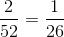### Example Question #24 : Calculating Probability

I have six black balls, four green balls, eight blue balls, and two red balls. What is the probability I pick either a blue or black ball?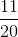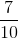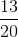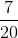Explanation:

Anytime we see the word either, this means we are adding probabilities.

We are looking for a blue or black ball.

Our total number of blue and black balls is fourteen.

The total number of balls is twenty.

So our probability is: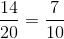### Example Question #25 : Calculating Probability

I have six black balls, four green balls, eight blue balls, and two red balls. What is the probability I pick a blue ball first and then a black ball without replacement?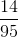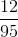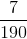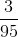Explanation:

Anytime we see the word and, we need to meet conditions. Therefore we need to multiply the probability.

The probability of getting a blue ball is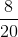or.

Next, the probability of getting a black ball is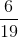because it says without replacement, this means by drawing a black ball, we only haveballs left.

Now, we multiply the fractions.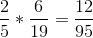### Example Question #26 : Calculating Probability

What is the probability of rolling aon a die?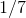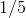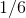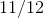Explanation:

Step 1: Find out how many numbers I can roll on a die.

I can rollnumbers: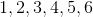.

Step 2: Find the probability of rolling a..

Each number on a die has a probability ofof being rolled.

### Example Question #27 : Calculating Probability

Find the probability of rolling a six on a fair die and then draw a spade in a standard 52- card deck.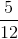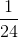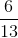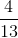Explanation:

A die has six faces with one number on each face.

The probability of rolling a six is:There are four suits in a standard 52 card deck.  There are 13 cards of a particular suit.   A spade consists of 13 of the 52 cards.  Write the probability of drawing a spade.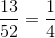Multiply the probabilities.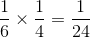The answer is:### Example Question #28 : Calculating Probability

Billy and Bob are tossing coins. Bob saw Billy tossed a fair coin six times, and the results were all heads.  Bob also wants to try.  What is the probability that Bob will toss heads twice in the next two coin tosses?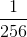Explanation:

Each coin toss is independent of one another.

The probability of landing on heads or tails is an even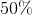, or, per trial.

For the next two coins rolling heads, Bob's probability of landing both heads is: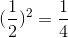The answer is:### Example Question #29 : Calculating Probability

Suppose a person tossed a fair coin 3 times.  What is the probability that the person will roll no heads in the process?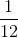Explanation:

Each toss of the fair coin will result in either heads or tails.

Write out all the combinations of the results for each of the coin tosses.

Let: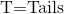The combinations are:

Three heads: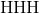Two heads: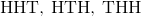One head: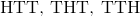No head: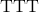Out of the eight possible combinations, only one of the possibilities have no heads.

The answer is:### Example Question #30 : Calculating Probability

Joe has two standard dice. Determine the probability that if he rolls them at the same time, he will roll a five and a one.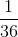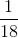None of theseExplanation:

The first die can either be a five or a one. Assuming a standard cubic dice, this would be a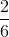orchance.

However, the second cube would need to be the remaining number. This is only aprobability .

Multiplying the two together: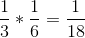This can be more easily visualized by drawing a map off all possibilities

### All Algebra II Resources